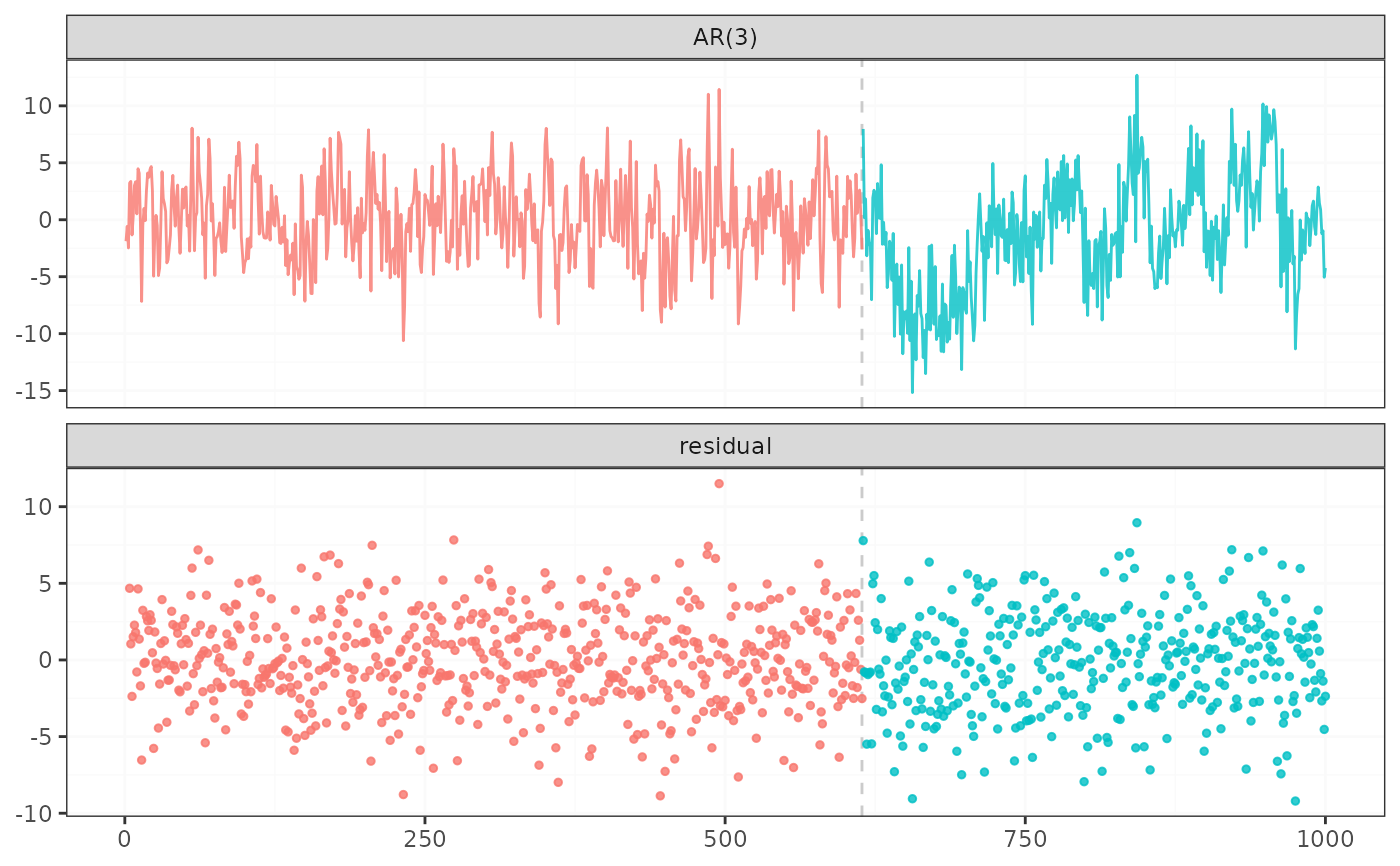fastcpd_ar and fastcpd.ar are wrapper functions of fastcpd to find change points in AR(p) models. The function is similar to fastcpd except that the data is by default a one-column matrix or univariate vector and thus a formula is not required here.

## Usage

fastcpd.ar(data, order = 0, ...)

fastcpd_ar(data, order = 0, ...)

## Arguments

data

A numeric vector, a matrix, a data frame or a time series object.

order

A positive integer or a vector of length three with the last two elements being zeros specifying the order of the AR model.

...

Other arguments passed to fastcpd, for example, segment_count. One special argument can be passed here is include.mean, which is a logical value indicating whether the mean should be included in the model. The default value is TRUE.

## Value

A class fastcpd object.

## Examples

set.seed(1)
n <- 1000
x <- rep(0, n + 3)
for (i in 1:600) {
x[i + 3] <- 0.6 * x[i + 2] - 0.2 * x[i + 1] + 0.1 * x[i] + rnorm(1, 0, 3)
}
for (i in 601:1000) {
x[i + 3] <- 0.3 * x[i + 2] + 0.4 * x[i + 1] + 0.2 * x[i] + rnorm(1, 0, 3)
}
result <- fastcpd.ar(x[3 + seq_len(n)], 3)
summary(result)
#>
#> Call:
#> fastcpd.ar(data = x[3 + seq_len(n)], order = 3)
#>
#> Change points:
#> 626
#>
#> Cost values:
#> 2857.934 1931.978
#>
#> Parameters:
#>    segment 1 segment 2
#> 1  0.5483503 0.2368461
#> 2 -0.1850633 0.4035386
#> 3  0.0666261 0.2352056
plot(result)